Definitions of Square Dance Calls and Concepts

Trapezoid (\$BBf7A(B) \$B\$O(B Distorted (\$B\$f\$,\$s\$@(B) 2 x 2 \$B\$G(B, \$BIaDL\$N(B 2 x 2 \$B\$+\$iNY\$j9g\$C\$??M\$N\$I\$A\$i\$+\$,(B, \$B\$*8_\$\$\$KN%\$l\$k\$h\$&\$K(B 1 \$B%]%8%7%g%sF0\$\$\$?\$H\$-\$K\$G\$-\$^\$9(B.

2 x 4 \$B\$+\$i(B, 2 \$B\$D\$N(B Trapezoid \$B\$,\$"\$j\$^\$9(B. Ends \$B\$O(B, \$BH?BPB&\$N(B Centers \$B\$H(B Distorted Box \$B\$GF0\$-\$^\$9(B.

Trapezoid Formation: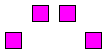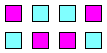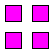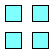\$B\$3\$3\$+\$i(B... \$B\$3\$3\$K\$\$\$k\$H;W\$C\$F(B... \$B\$^\$?\$O\$3\$3(B.

\$BCm
• \$B\$"\$k(B Trapezoid \$B\$N%3!<%k\$NDL\$jJ}\$O(B, \$B\$H\$s\$G\$b\$J\$\$\$b\$N\$K\$J\$j\$^\$9(B. \$BI,MW\$J\$i(B Star \$B\$r;H\$C\$F(B, \$BB>\$N?M\$H\$V\$D\$+\$i\$J\$\$\$h\$&\$K\$7\$F(B, \$B<+J,\$N=*\$o\$k>l=j\$X9T\$/\$h\$&\$K\$7\$F\$/\$@\$5\$\$(B.
• \$B%3!<%k\$G\$O(B, \$B%*%j%8%J%k\$N(B Trapezoid \$B\$N>l=j\$G=*\$o\$kI,MW\$,\$"\$j\$^\$9(B. Shape change \$B\$N%3!<%k\$O(B, \$B5v\$5\$l\$^\$;\$s(B.
• Trapezoid Couple Up: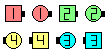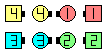Trapezoid Couple Up\$B\$NA0(B \$B8e(B

Once Removed Trapezoid Counter Rotate 1/4:
(\$B\$3\$l\$O(B, C4 \$B\$G\$"\$C\$F\$b(B, \$B\$H\$F\$bFq\$7\$\$\$G\$9(B)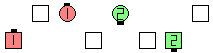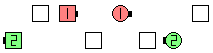Once Removed TrapezoidCounter Rotate 1/4\$B\$NA0(B \$B8e(B

\$BMagic Line | Wave CONCEPT [C3B] Magic \$B\$G\$O(B, \$BJ?9T\$J(B Line \$B\$+\$iF1\$80LCV\$GF0\$-\$^\$9\$,(B, Distorted Box \$B\$G\$J\$/(B Distorted Line \$B\$GF0\$-\$^\$9(B.